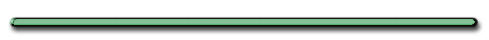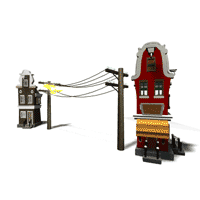Flowers in Liquid NitrogenEnergy
- Capacity to do workLevers give you a 'mechanical advantage'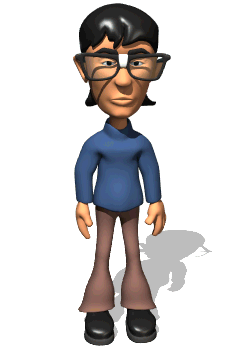Work always involves some tradeoff. Name 2 ways 1st gear is different than 10th gear.1st gear requires

a small force and a lot pedaling,

while 10th gear requires

little pedaling but  larger force.An Incline Plane is a Simple Machine

I) Work - Product of F and displacement in which force acts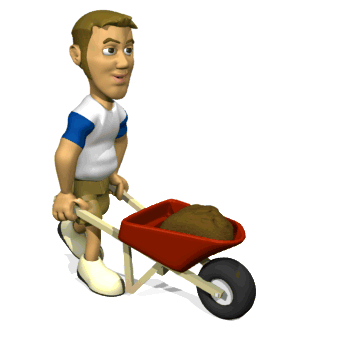Work and Energy

**Scalar**

A) Equation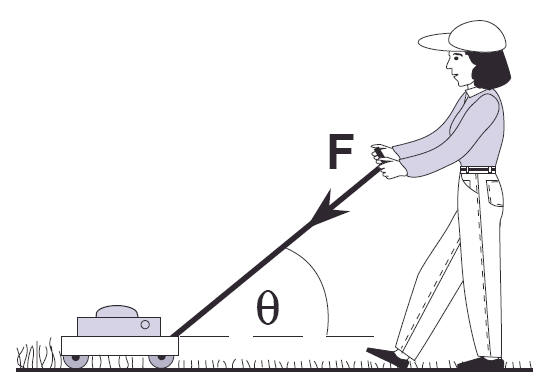W done by a specific force on an object

 W = Fcosq Δd (Nm) (N) (m)F = Force

Δd - displacement

Ө = angle between F and d

Other units for work (Joule) or Kg m2/sec2If you have Multiple Forces

 Wnet = Fnetcosq Δd (Nm) (N) (m)

Work done by all the forces on object

Finding Wnet
W and F +

if acting
in same direction as d

(Pushing on lawnmower)Finding Fnet
W and F negative
if acting against d

(A car braking, friction, catching baseball)

If Ө between
F and d   = 90

W = 0Ex) Object pushed at angle Ө, f=0, mass M, Force F, distance dW = Fcosq Δd (Nm) (N) (m)Ex) Object pushed at angle, f≠0, Mass M, Force F, μ, Fn

Find Net Work done on mowerWnet = Fcosqd - μFnd

Wnet = work by mower - work against

Why is Wf negative?

When finding Wnet
Wfriction = negative
Acts in the direction OPPOSITE d

Challenge Question: Two people exert a 10 Newton force on a block for 2 meters.  One person exerts her force at a 50-degree angle. (frictionless)

 Person 1 Person 2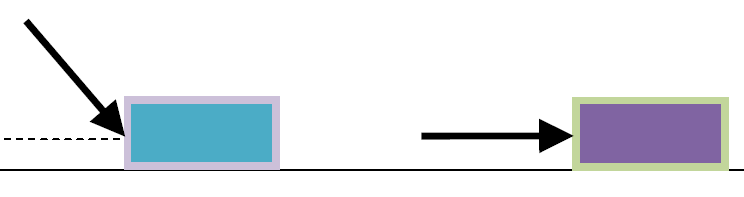Did both people do the same work???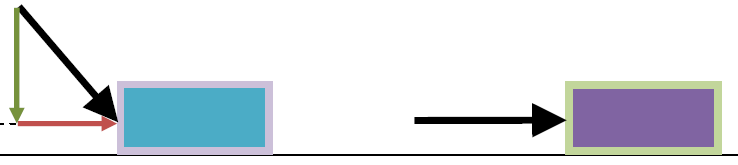Person 2 does more work
All of her force goes to
moving the block"Do the young man's HANDS do any work on the books he's holding?""No. The books do not move in the direction of the hand's force. (up) His legs do the work"Ex) Find minimum work needed to push a 950 kg car 810 m up a 9.0 degree incline,

a) no friction b) friction μ = 0.25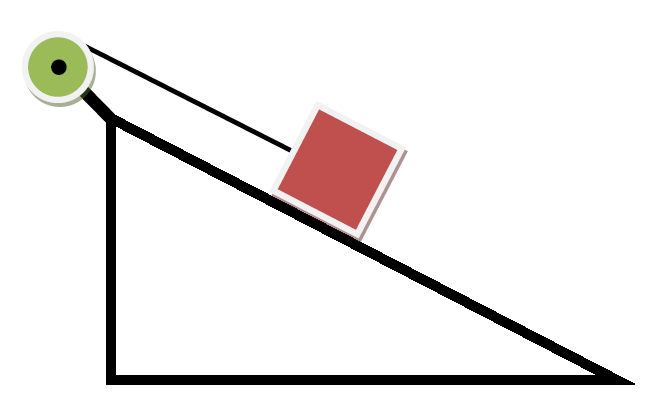Work is minimum
when Fnet = 0

W = [mgsinq]d

W = [950 kg x 9.8m/s2]sin9]810m

1.2 x 106 J

b) Find minimum work needed to push a 950 kg car 810 m up a 9.0 degree incline, friction μ = 0.25

Caution: We are not finding net work on box. We are finding the min. work to move box up incline.W = [mg(sinӨ+μcosӨ]d

= 3.0 x 106 J

 W = F Δd (Nm) (N) (m)

Textbook p. 162) 8

8.

330 kg piano, slides down 3.6 m, 28° incline

uk = .40

keep from accel. down ramp by man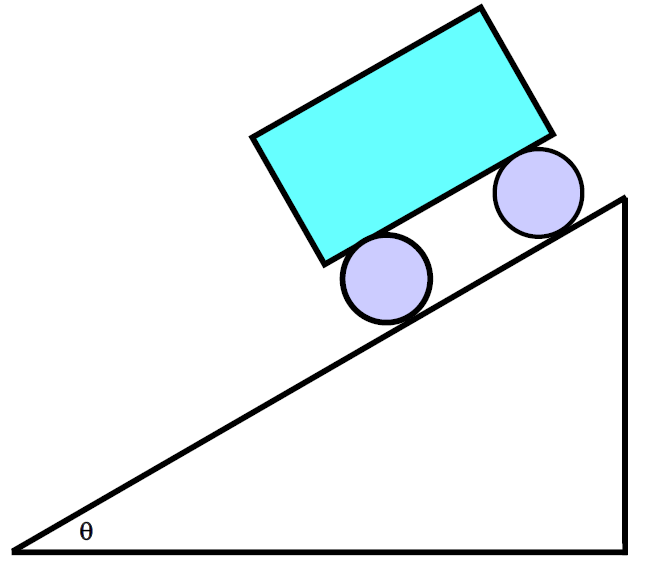(a) Force exerted by man?

Since a = 0 so Fnet = 0 down ramp

Free body Diagram on piano

Fdown = Fup

mgsinӨ = fk + Fman

mgsinӨ = ukFn + Fman

mgsinӨ = ukmgcosӨ + Fman

mgsinӨ - ukmgcosӨ = Fman

Fman = 3.8 x 102 N

b) Work done by man on piano?

Work + or - ?

 W = F Δd (Nm) (N) (m)

W = -3.8 x 102 N(3.6m)

= -1.4 x 103 J

c) Work done by friction

Work + or -?

 W = F Δd (Nm) (N) (m)

Wf = -[ukFn]d

= - ukmgcosӨ[d]

Wf = -4.1 x 103 J

d) Work done by force of gravity?

Work + or -?

W = mgsinӨ[d]

5.5 x 103 J

e) Net work done on piano?

Wnet = Fnetd

Since constant v then a  = 0 then

Fnet = 0

W = 0

Or

Wnet = Wman + Wfr + Wg

Wnet = Wman + Wfr + Wg

2 forces opp. direction of d, one F with d

Wnet = -1400 J + -4100J + 5500 J

Wnet = 0 JB) Work and F vs. d diagram (plot)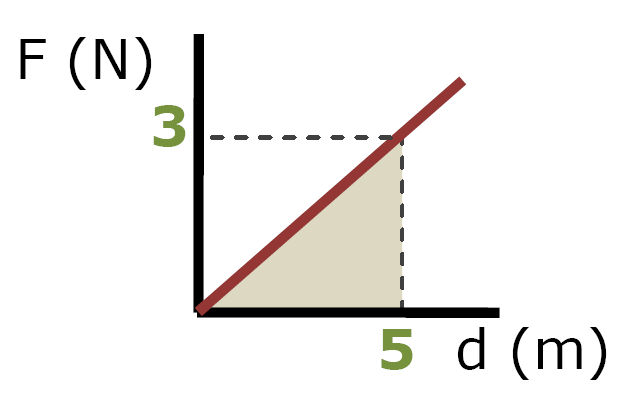Work = Area under a Force vs. d plot

W = Area Under Plot

= 1/2bh

= 1/2(5 m)(3 N)

7.5 Nm

p. 162) 14

• F increases linearly from
x = 0, F = 0 to x = 3.0 m F = 24 m

• F constant from x = 3.0 m to x = 8.0 m

• Then decreases linearly to 0 at x = 13.0 m

Sketch GraphArea under plot = W

W = 1/2[b1 + b2]h

W = 1/2[13m+5m]24 N

W = 216 J